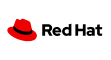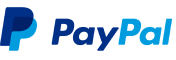New update is available. Click here to update.
Topics

# K Largest Element

Moderate0/80
Average time to solve is 10m+21 more companies

## Problem statement

You are given an unsorted array containing ‘N’ integers. You need to find ‘K’ largest elements from the given array. Also, you need to return the elements in non-decreasing order.

Detailed explanation ( Input/output format, Notes, Images )
Constraints:
``````1 <= T <= 100
1 <= N <= 10^4
1<= K <= N
-10^9 <= ARR[i] <= 10^9

Where ‘T’ is the number of test cases, ‘N’ is the size of the array, ‘K’ is the number of elements you need to return as an answer and ARR[i] is the size of the array of elements.

Time Limit: 1 sec
``````
Sample Input 1:
``````2
4 2
3 4 2 1
5 1
2 2 3 3 1
``````
Sample Output 1:
``````3 4
3
``````
Explanation for sample input 1:
``````Test case 1:
If we sort the array then it will look like: [1, 2, 3, 4]. The 2 largest elements will be [3, 4].

Test case 2:
If we sort the array then it will look like: [1, 2, 2, 3, 3]. Then the largest element will be .
``````
Sample Input 2:
``````2
5 5
0 10 1 2 2
6 2
-2 12 -1 1 20 1
``````
Sample Output 2:
``````0 1 2 2 10
12 20
``````Console